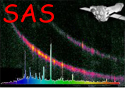XMM-Newton Science Analysis System

omdetect (omdetect-5.41) [xmmsas_20190531_1155-18.0.0]

# Output source-list file

The source-list file produced by omdetect contains a list of the positions of all the detected sources, the parameterizations of their shapes, their extracted count-rates, and "corrected count-rates" which contain some of the corrections that are needed to convert the raw rates to the rates that can be used to compute instrumental magnitutes. Fully corrected count-rates and instrumental magnitudes are obtained by running ommag, with its parameter “set” set to the omdetect source-list.

The source-list file is in FITS file format and contains a SRCLIST table with the columns shown in the following table.

KIND UNITS DESCRIPTION COLUMN NAME INT 32 none Source number - order of detection FLOAT pixels x-coordinate of centroided source centre FLOAT pixels y-coordinate of centroided source centre FLOAT pixels error in x and y centroided position DOUBLE degrees RA (J2000) mosaiced sky-images only DOUBLE degrees DEC (J2000) mosaiced sky-images only DOUBLE degrees RA (J2000) corrected for the boresight offset DOUBLE degrees DEC (J2000) corrected for the boresight offset DOUBLE degrees Galactic longitude DOUBLE degrees Galactic lattitude FLOAT counts/sec Total measured source count-rate (after background subtraction) within source extraction aperture (default aperture=12 un-binned pixels, but a smaller one may have been used), divided by exposure time. FLOAT counts/sec Error in the raw count-rate FLOAT counts/sec Total estimated background count-rate (scaled by area ratio, from the background annulus) within default source extraction aperture, divided by exposure time FLOAT none source counts / sqrt(source + background counts) i.e. signal-to-noise ratio, where the counts refer to the rawcounts, before any corrections have been applied. FLOAT counts/sec Background subtracted source count rate after applying PSF (if necessary) and coincidence-loss corrections. FLOAT counts/sec Error in the corrected rate FLOAT counts/sec BACKGROUND_RATE after correcting for coincidence losses. FLOAT % Point-spread function correction applied to extrapolate the RATE value from the measurement aperture area to the default extraction area of 12 unbinned pixels FLOAT % Point-spread function correction applied to extrapolate the RATE value from the extraction area of 12 unbinned pixels to the CAL (photometric) radius FLOAT % Coincidence-loss correction applied to the source plus background rate (ratio of the coincidence-loss corrected source + coincincidence-loss corrected background count-rate to the raw source + raw background count-rate) FLOAT % Coincidence-loss correction applied to the raw-background rate (ratio of the coincidence-loss corrected background count-rate to the raw background count-rate) FLOAT mag Instrumental magnitude FLOAT mag Instrumental magnitude error INT 32 none Number of pixels contained by an extended source (EFLAG=1). Point-sources (EFLAG=0) will have this value set to zero. FLOAT unbinned pixels For point sources (EFLAG=0), the aperture radius (unbinned pixels) used in the photometry. For extended-sources (EFLAG=1) this value will be set to NULL.

KIND UNITS DESCRIPTION COLUMN NAME FLOAT arcsecs The full-width-at-half-maximum of the semi-major axis of the ellipse describing the shape of the source (computed by moments - Set to NULL if a reliable value couldn't be computed.) FLOAT pixels The computed error of the major-axis FLOAT pixels The full-width-at-half-maximum of the semi-minor axis of the ellipse describing the shape of the source (computed by moments - Set to NULL if a reliable value couldn't be computed.) FLOAT arcsecs The computed error of the minor-axis FLOAT degrees The position-angle (measured anti-clockwise from the positive x-axis) of the semi-major axis of the ellipse describing the shape of the source (computed from moments - Set to NULL if a reliable value couldn't be computed.) FLOAT degrees The computed error of the position angle INT 16 none Source quality flag - Please see below INT 8 none Source confusion flag - Please see below INT 8 none (source extension flag) Either 0 point-source (non-extended) or 1 extended. INT 32 none Source ID number in the source-list file

Subsections
XMM-Newton SOC/SSC -- 2019-06-02